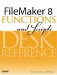# Int()

Category: Number

 Syntax: Int ( number )

Parameters:

numberAny expression that resolves to a numeric value.

Data type returned: Number

Description:

Returns the whole number (integer) part of the number parameter without rounding. Digits to the right of the decimal point are dropped.

Note that for positive numbers, Floor() and Int() return the same results; however, for negative numbers, Int() returns the next larger integer, whereas Floor() returns the next smaller integer.

There are many practical uses for the Int() function. For instance, given any date, to find the date of the Sunday preceding it, use the formula GetAsDate (Int (myDate/7) * 7). Similarly, to test whether an integer is odd or even, you can test whether Int (num/2) = num/2.

Examples:

Function

Results

Int (1.0005)

Returns 1.

Int (-1.0005)

Returns -1.FileMaker 8 Functions and Scripts Desk Reference
ISBN: 0789735113
EAN: 2147483647
Year: 2004
Pages: 352